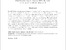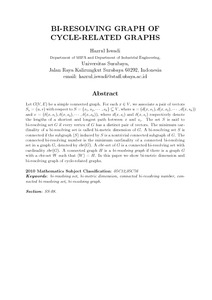# BI-RESOLVING GRAPH OF CYCLE-RELATED GRAPHS

Iswadi, Hazrul (2016) BI-RESOLVING GRAPH OF CYCLE-RELATED GRAPHS. In: The Asian Mathematical Conference 2016 (AMC 2016), July 25-29,2016, Bali. (Unpublished)Preview PDF AMC 2016 Hazrul.pdf Download (36kB) | Preview

## Abstract

Let $G(V,E)$ be a simple connected graph. For each $x \in V$, we associate a pair of vectors $S_x = (u,v)$ with respect to $S = \{s_1, s_2, \cdots, s_k\} \subseteq V$, where $u = (d(x,s_1), d(x,s_2), \cdots, d(x,s_k))$ and $v = (\delta(x,s_1), \delta(x,s_2), \cdots, \delta(x,s_k))$, where $d(x,s_i)$ and $\delta(x,s_i)$ respectively denote the lengths of a shortest and longest path between $x$ and $s_i$. The set $S$ is said to bi-resolving set $G$ if every vertex of $G$ has a distinct pair of vectors. The minimum cardinality of a bi-resolving set is called bi-metric dimension of $G$. A bi-resolving set $S$ is connected if the subgraph $\langle S \rangle$ induced by $S$ is a nontrivial connected subgraph of $G$. The connected bi-resolving number is the minimum cardinality of a connected bi-resolving set in a graph $G$, denoted by $cbr(G)$. A \textit{cbr}-set of $G$ is a connected bi-resolving set with cardinality $cbr(G)$. A connected graph $H$ is a \textit{bi-resolving graph} if there is a graph $G$ with a cbr-set $W$ such that $\langle W \rangle = H$. In this paper we show bi-metric dimension and bi-resolving graph of cycle-related graphs.

Item Type: Conference or Workshop Item (Poster) bi-resolving set, bi-metric dimension, connected bi-reso lving number, connected bi-resolving set, bi-resolving graph Q Science > QA Mathematics Academic Department > Department of Mathematics and Natural Science Hazrul Iswadi 6179 04 Jul 2018 04:02 24 Mar 2021 15:50 http://repository.ubaya.ac.id/id/eprint/32609View Item